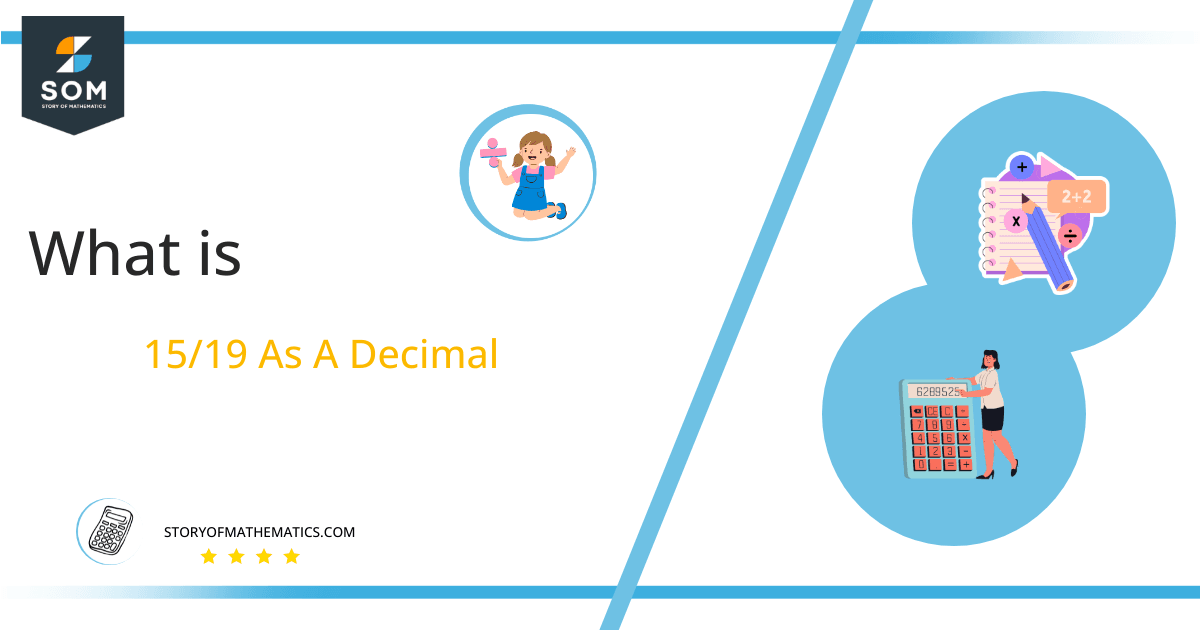# What Is 15/19 as a Decimal + Solution With Free Steps

The fraction 15/19 as a decimal is equal to 0.789.

A Fractional number requires to be converted into a decimal form in mathematical situations. The long division method is used to convert the fractional value where the numerator becomes the dividend and the denominator becomes the divisor and the quotient is the decimal form that is needed.

Here, we are more interested in the division types that result in a Decimal value, as this can be expressed as a Fraction. We see fractions as a way of showing two numbers having the operation of Division between them that result in a value that lies between two Integers.Now, we introduce the method used to solve said fraction to decimal conversion, called Long Division, which we will discuss in detail moving forward. So, let’s go through the Solution of fraction 15/19.

## Solution

First, we convert the fraction components, i.e., the numerator and the denominator, and transform them into the division constituents, i.e., the Dividend and the Divisor, respectively.

This can be done as follows:

Dividend = 15

Divisor = 19

Now, we introduce the most important quantity in our division process: the Quotient. The value represents the Solution to our division and can be expressed as having the following relationship with the Division constituents:

Quotient = Dividend $\div$ Divisor = 15 $\div$ 19

This is when we go through the Long Division solution to our problem. Given is the Long division method in Figure 1:Figure 1

## 15/19 Long Division Method

We start solving a problem using the Long Division Method by first taking apart the division’s components and comparing them. As we have 15 and 19, we can see how 15 is Smaller than 19, and to solve this division, we require that 15 be Bigger than 19.

This is done by multiplying the dividend by 10 and checking whether it is bigger than the divisor or not. If so, we calculate the Multiple of the divisor closest to the dividend and subtract it from the Dividend. This produces the Remainder, which we then use as the dividend later.

Now, we begin solving for our dividend 15, which after getting multiplied by 10 becomes 150.

We take this 150 and divide it by 19; this can be done as follows:

150 $\div$ 19 $\approx$ 7

Where:

19 x 7 = 133

This will lead to the generation of a Remainder equal to 150 – 133 = 17. Now this means we have to repeat the process by Converting the 17 into 170 and solving for that:

170 $\div$ 19 $\approx$ 8

Where:

19 x 8 = 152

This, therefore, produces another Remainder which is equal to 170 – 152 = 18. Now we must solve this problem to Third Decimal Place for accuracy, so we repeat the process with dividend 180.

180 $\div$ 19 $\approx$ 9

Where:

19 x 9 = 171

Finally, we have a Quotient generated after combining the three pieces of it as 0.789, with a Remainder equal to 9.Images/mathematical drawings are created with GeoGebra.